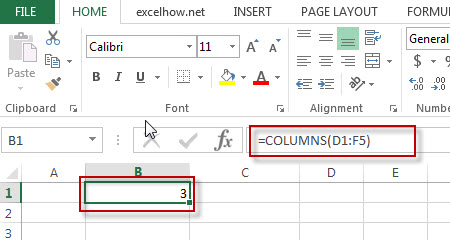# Excel Columns Function

This post will guide you how to use Excel COLUMNS function with syntax and examples in Microsoft excel.

### Description

The Excel COLUMNS function returns the number of columns in an Array or a reference.

The COLUMNS function is a build-in function in Microsoft Excel and it is categorized as a Lookup and Reference Function.

The COLUMNS function is available in Excel 2016, Excel 2013, Excel 2010, Excel 2007, Excel 2003, Excel XP, Excel 2000, Excel 2011 for Mac.

### Syntax

The syntax of the COLUMNS function is as below:

`=COLUMNS (array)`

Where the COLUMNS function arguments is:

• Array -This is a required argument.  A reference to a cell or a range of cells.

### Example

The below examples will show you how to use Excel COLUMNS Lookup and Reference Function to return the number of columns in a given range.

#1 To get the number of columns in the reference D1:F5, just using the following excel formula:

`=COLUMNS(D1:F5)`### More Excel COLUMNS Formula Examples

• Transpose Multiple Columns into One Column
You can use the following excel formula to transpose multiple columns that contain a range of data into a single column, and you can also write an Excel VBA Macro to transpose the data of range in B1:D4 into single column F quickly…
• Sum Specific Row or Column in Named Range
Assuming that you have a name range “excelhow”, if you want to sum a specific row (row 2) in named range, you need to create a formula based on the SUM function and the OFFSET function and the COLUMNS function to achieve the reuslt….
• Split Data in One Column to Multiple Columns
If you want to split each sets in one column from row to row in stacked columns, and you need to create a formula based on the OFFSET function, the COLUMNS function, and the ROWS function..…